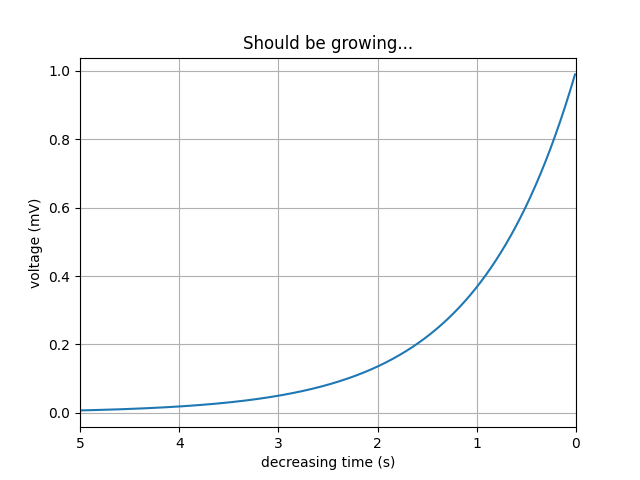# Invert Axes¶

You can use decreasing axes by flipping the normal order of the axis limitsimport matplotlib.pyplot as plt
import numpy as np

t = np.arange(0.01, 5.0, 0.01)
s = np.exp(-t)

fig, ax = plt.subplots()

ax.plot(t, s)
ax.set_xlim(5, 0)  # decreasing time
ax.set_xlabel('decreasing time (s)')
ax.set_ylabel('voltage (mV)')
ax.set_title('Should be growing...')
ax.grid(True)

plt.show()


Keywords: matplotlib code example, codex, python plot, pyplot Gallery generated by Sphinx-Gallery